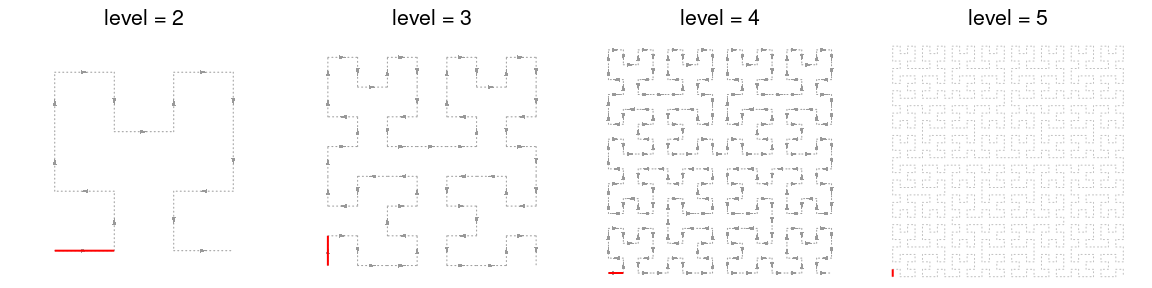# Making 2D Hilbert Curve

## Introduction

Hilbert curve is a type of space-filling curves that folds one dimensional axis into a two dimensional space, but still keeps the locality. It has advantages to visualize data with long axis in following two aspects:

1. greatly improve resolution of the visualization fron $$n$$ to $$\sqrt{n}$$;
2. easy to visualize clusters because generally data points in the axis will also be close in the 2D space.

This package aims to provide an easy and flexible way to visualize data through Hilbert curve. The implementation and example figures are based on following sources:

We first load the packages and set seed for random numbers.

library(HilbertCurve)
library(circlize)
set.seed(12345)

Following plots show Hilbert curves with level 2, 3, 4, 5:

## Warning in matrix(c(cos(theta), sin(theta), -sin(theta), cos(theta)), 2, : data length differs from
## size of matrix: [60 != 2 x 2]
## Warning in matrix(c(cos(theta), sin(theta), -sin(theta), cos(theta)), 2, : data length differs from
## size of matrix: [252 != 2 x 2]
## Warning in matrix(c(cos(theta), sin(theta), -sin(theta), cos(theta)), 2, : data length differs from
## size of matrix: [1020 != 2 x 2]As shown in the above plots, as level increases, the length of the curve becomes longer and the curve folds more densely. The number of segments (one segment is marked in red) on the Hilbert curve is 4^level - 1. If a Hilbert curve with level 11 is used to map to human chromosome 1, the resolution would be 249250621/4^11 (approximately 59bp per segment).

### Locality

Hilbert curve folds one-dimensional axis into a two-dimensional space while still preserves the locality of the data points. We visualize this attribute with a Hilbert curve with level 5. In following animation, the point moves with its natural order on the axis.

for(i in 1:1024) {
hc = HilbertCurve(1, 1024, level = 5, reference = TRUE, arrow = FALSE)
hc_points(hc, x1 = i, np = NULL, pch = 16, size = unit(2, "mm"))
}

Next, we calculate the pairwise distance between data points in the 2D space and visualize it as heatmap. The numbers on x-axis (top) and y-axis (left) illustrate the position of data points in the original 1D axis.

library(HilbertVis)
pos = HilbertVis::hilbertCurve(5)
mat = as.matrix(dist(pos))
library(ComplexHeatmap)

ht = Heatmap(mat, name = "dist", cluster_rows = FALSE, cluster_columns = FALSE,
show_row_names = FALSE, show_column_names = FALSE,
heatmap_legend_param = list(title = "euclidean_dist"))
draw(ht, padding = unit(c(5, 5, 5, 2), "mm"))
decorate_heatmap_body("dist", {
grid.segments(c(0.25, 0.5, 0.75, 0, 0, 0), c(0, 0, 0, 0.25, 0.5, 0.75),
c(0.25, 0.5, 0.75, 1, 1, 1), c(1, 1, 1, 0.25, 0.5, 0.75), gp = gpar(lty = 2))
grid.text(rev(c(256, 512, 768, 1024)), 0, c(0, 256, 512, 768)/1024, just = "bottom",
rot = 90, gp = gpar(fontsize = 10))
grid.text(c(1, 256, 512, 768, 1024), c(1, 256, 512, 768, 1024)/1024, 1, just = "bottom",
gp = gpar(fontsize = 10))
})